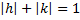# If sum of the distance of a point from two perpendicular lines in a plane is 1, then its locus is a) A square b) A circle c) A straight line d) Two intersecting lines

## Question ID - 52901 :- If sum of the distance of a point from two perpendicular lines in a plane is 1, then its locus is a) A square b) A circle c) A straight line d) Two intersecting lines

3537

(a)

Let the two perpendicular lines be taken as the coordinate axes. Ifbe any point on the locus, then according to the given condition. Hence, the locus ofis. This consists of four line segments enclosingsquare as shown in the figure belowNext Question :(whereare natural numbers) is any point in the intertior of the quadrilateral formed by the pair of linesand the linesand. The possible number of positions of the pointis a) 7 b) 5 c) 4 d) 6